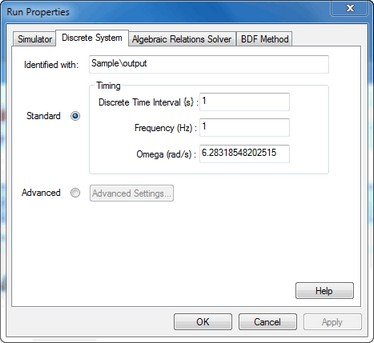﻿ 20-sim webhelp > Simulator > Run Properties > Discrete System Tab

# Discrete System Tab

The Run Properties Editor can be used to edit the sample frequency of a discrete-time system in the model. 20-sim automatically detects the existence of discrete systems in a model. For each discrete system, one extra tab (Discrete System) is shown in theThe Discrete Systems tab.

## Items

 • Identified with: Each discrete system in a model is assigned a unique identifier. 20-sim detects discrete systems by looking for discrete functions and variables:

 1 sample
 2 hold
 3 next
 4 previous
 5 sampletime

When a function has been found, the elements of the corresponding discrete system are identified by propagation of the equations. In order of appearance in the model, the discrete system is assigned a unique identifier:

 1 sample -> the variable that is assigned to the output of the sample function.
 2 hold -> the variable that is assigned to the output of the hold function.
 3 previous -> the discrete state corresponding with the previous function.
 4 next -> the discrete state corresponding with the next function.
 5 sampletime -> the variable that is equal to the sampletime.

 • Timing (Standard): Each discrete system has a default sample frequency of 1 Hz. You can change this frequency to any desired value:
 • Discrete Time Interval (s): The sample interval (in s) of the discrete system
 • Frequency (Hz): The sample frequency (in Hz) of the discrete system.
 • Timing (Advanced): For discrete-time control loops that interact with physical continuous-time systems through sensors (analog to digital) and actuators (digital to analog) the specific timing is important. Click the Advanced button to specify this timing.